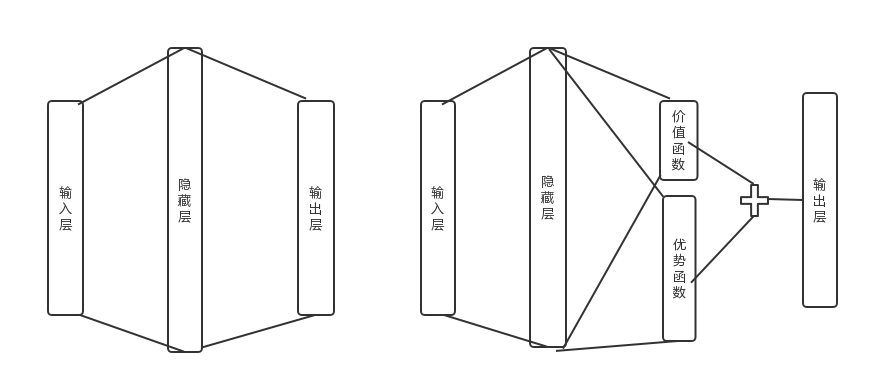# 强化学习(十二) Dueling DQN

在强化学习(十一) Prioritized Replay DQN中，我们讨论了对DQN的经验回放池按权重采样来优化DQN算法的方法，本文讨论另一种优化方法，Dueling DQN。本章内容主要参考了ICML 2016的deep RL tutorial和Dueling DQN的论文<Dueling Network Architectures for Deep Reinforcement Learning>(ICML 2016)。

# 1. Dueling DQN的优化点考虑

在前面讲到的DDQN中，我们通过优化目标Q值的计算来优化算法，在Prioritized Replay DQN中，我们通过优化经验回放池按权重采样来优化算法。而在Dueling DQN中，我们尝试通过优化神经网络的结构来优化算法。

具体如何优化网络结构呢？Dueling DQN考虑将Q网络分成两部分，第一部分是仅仅与状态$S$有关，与具体要采用的动作$A$无关，这部分我们叫做价值函数部分，记做$V(S,w,\alpha)$,第二部分同时与状态状态$S$和动作$A$有关，这部分叫做优势函数(Advantage Function)部分,记为$A(S,A,w,\beta)$,那么最终我们的价值函数可以重新表示为：$$Q(S,A, w, \alpha, \beta) = V(S,w,\alpha) + A(S,A,w,\beta)$$

其中，$w$是公共部分的网络参数，而$\alpha$是价值函数独有部分的网络参数，而$\beta$是优势函数独有部分的网络参数。

# 2. Dueling DQN网络结构

由于Q网络的价值函数被分为两部分，因此Dueling DQN的网络结构也和之前的DQN不同。为了简化算法描述，这里不使用原论文的CNN网络结构，而是使用前面文中用到的最简单的三层神经网络来描述。是否使用CNN对Dueling DQN算法本身无影响。

在前面讲到的DDQN等DQN算法中，我使用了一个简单的三层神经网络：一个输入层，一个隐藏层和一个输出层。如下左图所示：而在Dueling DQN中，我们在后面加了两个子网络结构，分别对应上面上到价格函数网络部分和优势函数网络部分。对应上面右图所示。最终Q网络的输出由价格函数网络的输出和优势函数网络的输出线性组合得到。

我们可以直接使用上一节的价值函数的组合公式得到我们的动作价值，但是这个式子无法辨识最终输出里面$V(S,w,\alpha)$和$A(S,A,w,\beta)$各自的作用，为了可以体现这种可辨识性(identifiability),实际使用的组合公式如下：$$Q(S,A, w, \alpha, \beta) = V(S,w,\alpha) + (A(S,A,w,\beta) - \frac{1}{\mathcal{A}}\sum\limits_{a' \in \mathcal{A}}A(S,a', w,\beta))$$

其实就是对优势函数部分做了中心化的处理。以上就是Duel DQN的主要算法思路。由于它仅仅涉及神经网络的中间结构的改进，现有的DQN算法可以在使用Duel DQN网络结构的基础上继续使用现有的算法。由于算法主流程和其他算法没有差异，这里就不单独讲Duel DQN的算法流程了。

# 3. Dueling DQN实例

下面我们用一个具体的例子来演示Dueling DQN的应用。仍然使用了OpenAI Gym中的CartPole-v0游戏来作为我们算法应用。CartPole-v0游戏的介绍参见这里。它比较简单，基本要求就是控制下面的cart移动使连接在上面的pole保持垂直不倒。这个任务只有两个离散动作，要么向左用力，要么向右用力。而state状态就是这个cart的位置和速度， pole的角度和角速度，4维的特征。坚持到200分的奖励则为过关。

这个实例代基于Nature DQN，并将网络结构改为上图中右边的Dueling DQN网络结构，完整的代码参见我的github: https://github.com/ljpzzz/machinelearning/blob/master/reinforcement-learning/duel_dqn.py

这里我们重点关注Dueling DQN和Nature DQN的代码的不同之处。也就是网络结构定义部分，主要的代码如下，一共有两个相同结构的Q网络，每个Q网络都有状态函数和优势函数的定义，以及组合后的Q网络输出，如代码红色部分：

  def create_Q_network(self):
# input layer
self.state_input = tf.placeholder("float", [None, self.state_dim])
# network weights
with tf.variable_scope('current_net'):
W1 = self.weight_variable([self.state_dim,20])
b1 = self.bias_variable()

# hidden layer 1
h_layer_1 = tf.nn.relu(tf.matmul(self.state_input,W1) + b1)

# hidden layer  for state value
with tf.variable_scope('Value'):
W21= self.weight_variable([20,1])
b21 = self.bias_variable()
self.V = tf.matmul(h_layer_1, W21) + b21

# hidden layer  for action value
W22 = self.weight_variable([20,self.action_dim])
b22 = self.bias_variable([self.action_dim])
self.A = tf.matmul(h_layer_1, W22) + b22

# Q Value layer
self.Q_value = self.V + (self.A - tf.reduce_mean(self.A, axis=1, keep_dims=True))

with tf.variable_scope('target_net'):
W1t = self.weight_variable([self.state_dim,20])
b1t = self.bias_variable()

# hidden layer 1
h_layer_1t = tf.nn.relu(tf.matmul(self.state_input,W1t) + b1t)

# hidden layer  for state value
with tf.variable_scope('Value'):
W2v = self.weight_variable([20,1])
b2v = self.bias_variable()
self.VT = tf.matmul(h_layer_1t, W2v) + b2v

# hidden layer  for action value
W2a = self.weight_variable([20,self.action_dim])
b2a = self.bias_variable([self.action_dim])
self.AT = tf.matmul(h_layer_1t, W2a) + b2a

# Q Value layer
self.target_Q_value = self.VT + (self.AT - tf.reduce_mean(self.AT, axis=1, keep_dims=True))

其余部分代码和Nature DQN基本相同。当然，我们可以也在前面DDQN，Prioritized Replay DQN代码的基础上，把网络结构改成上面的定义，这样Dueling DQN也可以起作用。

# 4. DQN总结

DQN系列我花了5篇来讲解，一共5个前后有关联的算法：DQN(NIPS2013), Nature DQN, DDQN, Prioritized Replay DQN和Dueling DQN。目前使用的比较主流的是后面三种算法思路，这三种算法思路也是可以混着一起使用的，相互并不排斥。

当然DQN家族的算法远远不止这些，还有一些其他的DQN算法我没有详细介绍，比如使用一些较复杂的CNN和RNN网络来提高DQN的表达能力，又比如改进探索状态空间的方法等，主要是在DQN的基础上持续优化。

DQN算是深度强化学习的中的主流流派，代表了Value-Based这一大类深度强化学习算法。但是它也有自己的一些问题，就是绝大多数DQN只能处理离散的动作集合，不能处理连续的动作集合。虽然NAF DQN可以解决这个问题，但是方法过于复杂了。而深度强化学习的另一个主流流派Policy-Based而可以较好的解决这个问题，从下一篇我们开始讨论Policy-Based深度强化学习。

（欢迎转载，转载请注明出处。欢迎沟通交流： liujianping-ok@163.com）

posted @ 2018-11-08 14:04  刘建平Pinard  阅读(32788)  评论(54编辑  收藏  举报